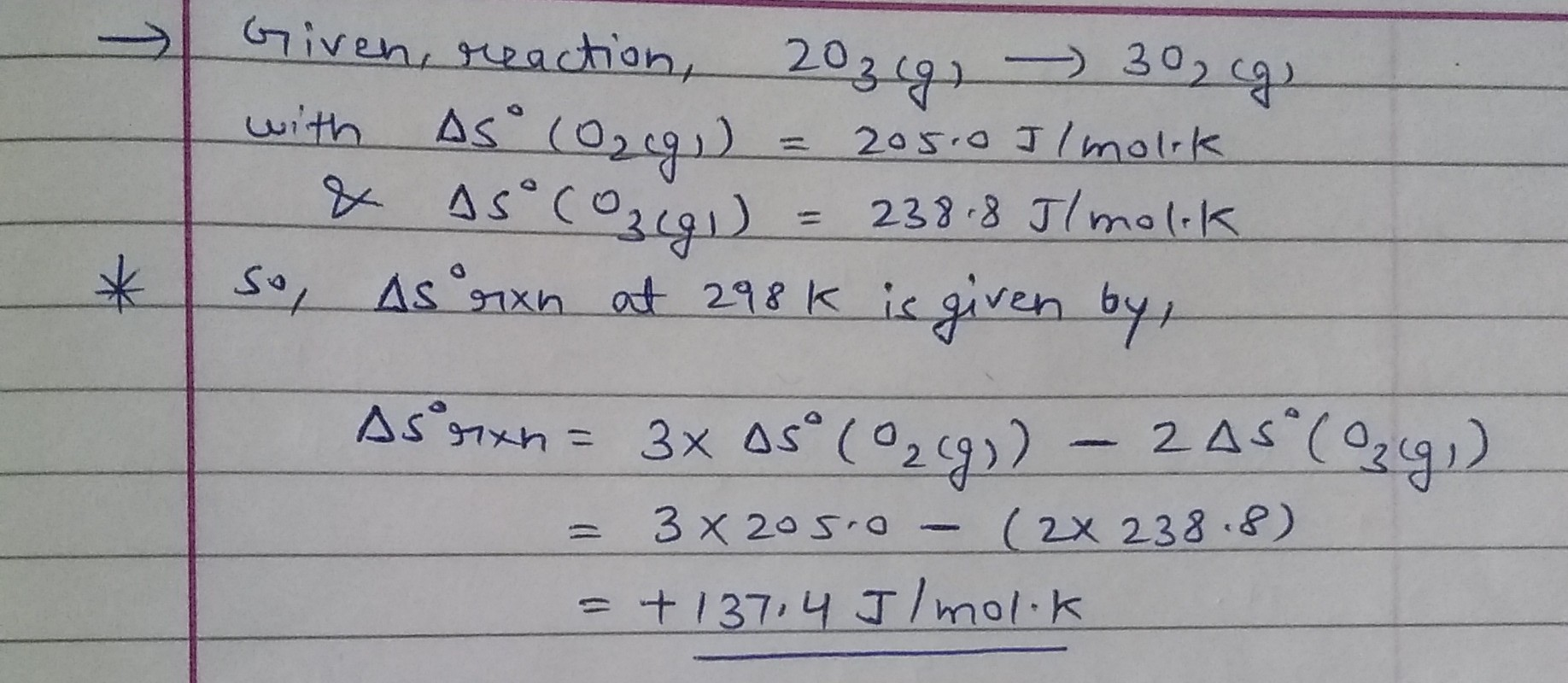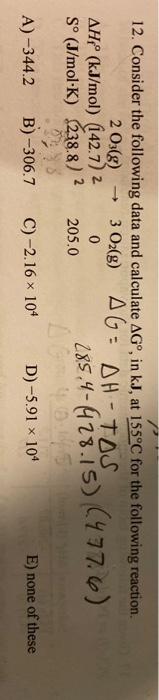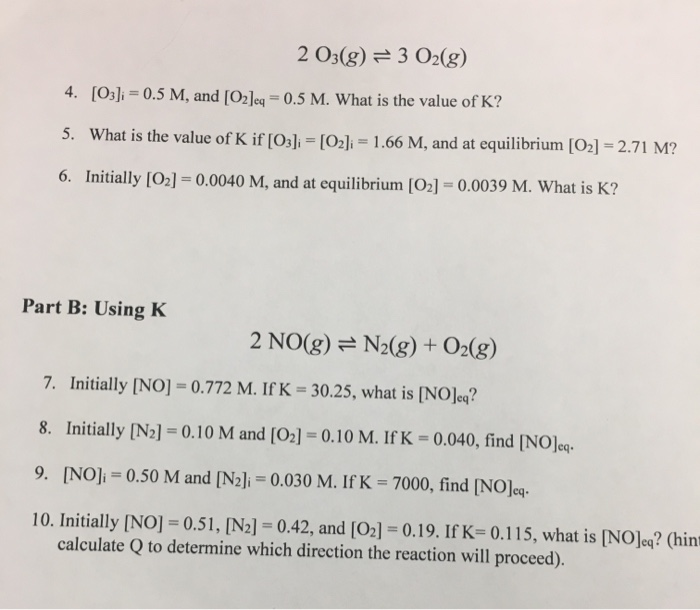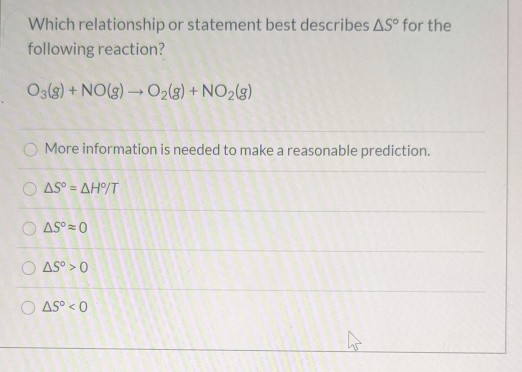Homework Help Question & Answers

# What is the ΔS° at 298 K for the following reaction? 2 O3(G) ---- 3 O2(G)...

What is the ΔS° at 298 K for the following reaction?

2 O3(G) ---- 3 O2(G)

 Substance ΔS° (J/mol·K) O2(g) 205.0 O3(g) 238.8

#### Homework Answers

Answer #1Know the answer?
Your Answer:

#### Post as a guest

Your Name:

What's your source?

#### Earn Coin

Coins can be redeemed for fabulous gifts.

Not the answer you're looking for? Ask your own homework help question. Our experts will answer your question WITHIN MINUTES for Free.
Similar Homework Help Questions
• ### the answer is A: -344.2 2 O3(g) AHP (kJ/mol) 42.7) 2 So (J/mol-K) 238.8) 2 12....the answer is A: -344.2 2 O3(g) AHP (kJ/mol) 42.7) 2 So (J/mol-K) 238.8) 2 12. Consider the following data and calculate AG°, in kJ, at 155°C for the following reaction. 3 O2(8) AG DH-TOS 0 N-1S77.6) 205.0 A)-344.2 B)-306.7 C)-2.16x 104 D)-5.91 x 104 E) none of these

• ### Calculate ΔS∘rxn for the reaction 2NO(g)+O2(g)→2NO2(g) Substance S∘ (J/mol⋅K) NO2 240.0 O2 205.2...

Calculate ΔS∘rxn for the reaction 2NO(g)+O2(g)→2NO2(g) Substance S∘ (J/mol⋅K) NO2 240.0 O2 205.2 NO 210.8

• ### For the values give for ΔH and ΔS, calculate ΔG foreach for each of the following reactions at 298 K. If the reactionis...

For the values give for ΔH and ΔS, calculate ΔG foreach for each of the following reactions at 298 K. If the reactionis not spontaneous under standard conditions at 298 K, at whattemperature (if any) would the reaction become spontaneous? a) 2PbS(s)+3O2(g) ---> 2PbO(s)+2SO2(g) ΔH= -844kj ; ΔS = -165 J/K b)2POCl3(g)--->2PCl3(g)+O2(g) ΔH= 572kJ ; ΔS= 179 J/K

• ### For the following reaction: 2 CO(g) + O2(g) → 2 CO2(g), given ΔH = -566 kJ and ΔG = -514 kJ, find ΔS (J/K) at 25 oC....

For the following reaction: 2 CO(g) + O2(g) → 2 CO2(g), given ΔH = -566 kJ and ΔG = -514 kJ, find ΔS (J/K) at 25 oC. Multiple Choices: ΔS = -174 J/K ΔS = -0.174 J/K ΔS = -2.08J/K ΔS = 174 J/K ΔS = -2080 J/K

• ### What is ∆So at 298 K for the following reaction? 2 H2S(g) + 3 O2(g) →...

What is ∆So at 298 K for the following reaction? 2 H2S(g) + 3 O2(g) → 2 SO2(g) + 2 H2O(g)    ΔH°f (kJ/mol)     -20.6 -296.8   -241.8 Δ G°f( kJ/mol) -33 -300.2 -228.60

• ### a) Calculate K at 298 K for the following reaction: NO(g) + 1 2 O2(g) ?...

a) Calculate K at 298 K for the following reaction: NO(g) + 1 2 O2(g) ? NO2(g) ?G0f(kj/mol) NO(g) 86.60 NO2(g) 51 O2(g) 0 K = ×10

• ### For the following reaction 3 O2 (g)   → 2 O3 (g)   ΔH = +286 kJ What...

For the following reaction 3 O2 (g)   → 2 O3 (g)   ΔH = +286 kJ What is the ΔH when 16.0 g of O3 are produced

• ### 2 O3(g) 3 O2(g) 4. [O3]i 0.5 M, and [O2]eq = 0.5 M. What is the...2 O3(g) 3 O2(g) 4. [O3]i 0.5 M, and [O2]eq = 0.5 M. What is the value of K? What is the value of K if [O3]i = [O2]i = 1.66 M, and at equilibrium [O2] 2.71 M? 5. 6. Initially [O2] = 0.0040 M, and at equilibrium [O2] = 0.0039 M. What is K? Part B: Using K 2 NO(g) N2(g) + O2(g) 7. Initially [NO] = 0.772 M. If K = 30.25, what is [NOJeq? 8. Initially [N2]...

• ### Which relationship or statement best describes AS for the following reaction? O3(g) + NO(g) + O2(g)...Which relationship or statement best describes AS for the following reaction? O3(g) + NO(g) + O2(g) + NO2(g) More information is needed to make a reasonable prediction. Ο ΔS = ΔΗ/T AS°=0 O AS'>0 O 45° < 0 W

• ### Ozone is formed from oxygen by the following reaction: 3/2 O2(g) ⇌ O3(g) Kp = 3.4...

Ozone is formed from oxygen by the following reaction: 3/2 O2(g) ⇌ O3(g) Kp = 3.4 x 10-38 at 220 K. Calculate the value of Kc.

Free Homework App

Scan Your Homework
to Get Instant Free Answers
Need Online Homework Help?

Get Answers For Free
Most questions answered within 3 hours.